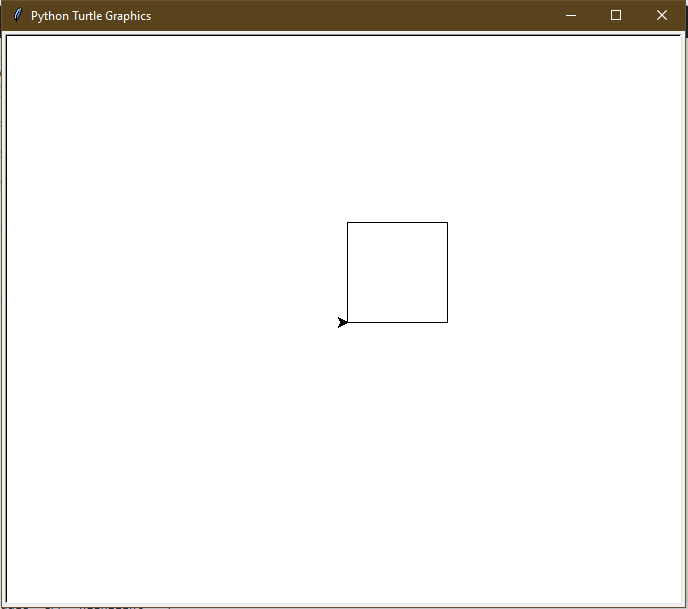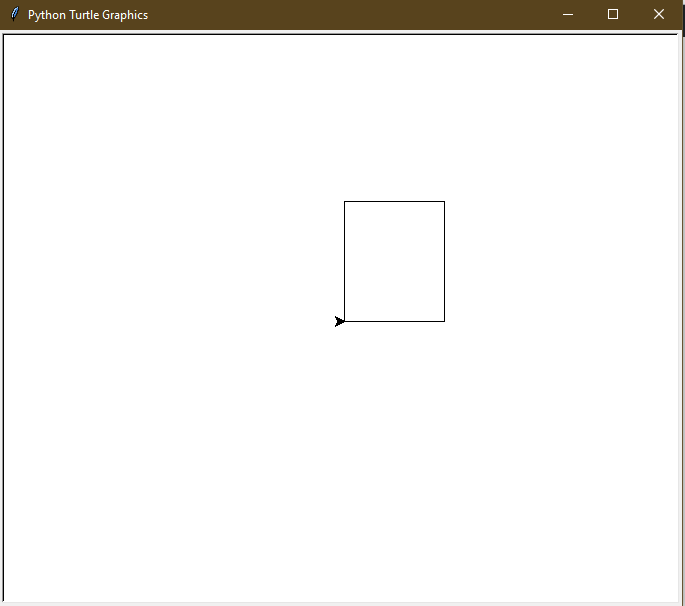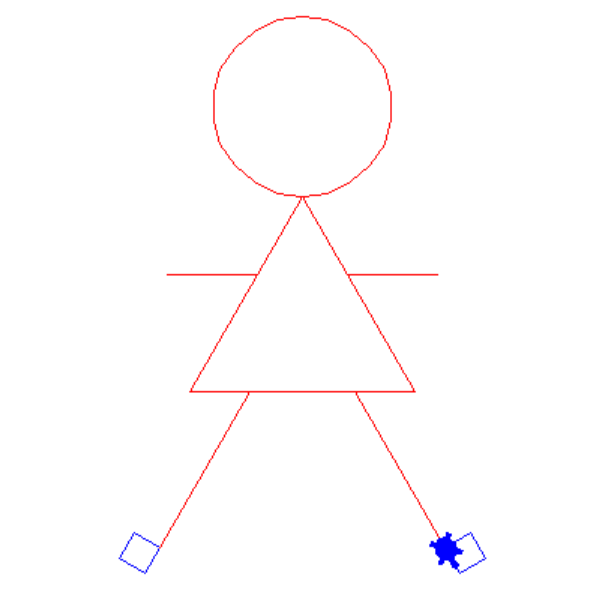GFG App
Open AppBrowser
Continue

# Draw Square and Rectangle in Turtle – Python

Prerequisite: Turtle Programming Basics
turtle is an inbuilt module in Python. It provides drawing using a screen (cardboard) and turtle (pen). To draw something on the screen, we need to move the turtle (pen). To move turtle, there are some functions i.e forward(), backward(), etc.

## Python3

 `# draw square in Python Turtle` `import` `turtle` ` `  `t ``=` `turtle.Turtle()`   `s ``=` `int``(``input``(``"Enter the length of the side of the Square: "``))`   `# drawing first side` `t.forward(s) ``# Forward turtle by s units` `t.left(``90``) ``# Turn turtle by 90 degree`   `# drawing second side` `t.forward(s) ``# Forward turtle by s units` `t.left(``90``) ``# Turn turtle by 90 degree`   `# drawing third side` `t.forward(s) ``# Forward turtle by s units` `t.left(``90``) ``# Turn turtle by 90 degree`   `# drawing fourth side` `t.forward(s) ``# Forward turtle by s units` `t.left(``90``) ``# Turn turtle by 90 degree`

Input :

`100`

Output :Second approach (Using Loop) :

## Python3

 `# draw Square in Python Turtle` `import` `turtle` ` `  `t ``=` `turtle.Turtle()`   `s ``=` `int``(``input``(``"Enter the length of the side of square: "``))`   `for` `_ ``in` `range``(``4``):` `  ``t.forward(s) ``# Forward turtle by s units` `  ``t.left(``90``) ``# Turn turtle by 90 degree`

Input :

`100`

Output :## Python3

 `# draw Rectangle in Python Turtle` `import` `turtle` ` `  `t ``=` `turtle.Turtle()`   `l ``=` `int``(``input``(``"Enter the length of the Rectangle: "``))` `w ``=` `int``(``input``(``"Enter the width of the Rectangle: "``))`   `# drawing first side` `t.forward(l) ``# Forward turtle by l units` `t.left(``90``) ``# Turn turtle by 90 degree`   `# drawing second side` `t.forward(w) ``# Forward turtle by w units` `t.left(``90``) ``# Turn turtle by 90 degree`   `# drawing third side` `t.forward(l) ``# Forward turtle by l units` `t.left(``90``) ``# Turn turtle by 90 degree`   `# drawing fourth side` `t.forward(w) ``# Forward turtle by w units` `t.left(``90``) ``# Turn turtle by 90 degree`

Input :

```100
120```

Output :Second approach (Using Loop) :

## Python3

 `# draw Rectangle in Python Turtle` `import` `turtle` ` `  `t ``=` `turtle.Turtle()`   `l ``=` `int``(``input``(``"Enter the length of the Rectangle: "``))` `w ``=` `int``(``input``(``"Enter the width of the Rectangle: "``))`   `for` `_ ``in` `range``(``4``):` `  `  `  ``# drawing length` `  ``if` `_``%` `2` `=``=` `0``:` `    ``t.forward(l) ``# Forward turtle by l units` `    ``t.left(``90``) ``# Turn turtle by 90 degree`   `  ``# drawing width` `  ``else``:` `    ``t.forward(w) ``# Forward turtle by w units` `    ``t.left(``90``) ``# Turn turtle by 90 degree`

Input :

```100
120```

Output :Since as of now, you must have learned how to draw various basic geometrical illustrations like circle, square, rectangle. So, let’s implement this knowledge to build something which you can really use in building games like let’s draw a human being using the basic knowledge of geometrical knowledge.

Here the code for this implementation:-

## Python3

 `import` `turtle`   `def` `draw_dream():` `    ``window ``=` `turtle.Screen()` `    ``window.bgcolor(``"white"``)` `    ``draw_Scarlett()` `    ``window.exitonclick()`     `def` `draw_Scarlett():` `    ``brad ``=` `turtle.Turtle()` `    ``brad.shape(``"turtle"``)` `    ``brad.color(``"red"``)` `    ``draw_head(brad)` `    ``draw_body(brad)` `    ``draw_arm(brad)` `    ``draw_leg1(brad)` `    ``draw_leg2(brad)`     `def` `draw_head(brad):` `    ``brad.circle(``60``)` `    ``brad.speed(``3``)` `    ``brad.right(``60``)`     `def` `draw_body(brad):` `    ``num ``=` `0` `    ``while` `num < ``3``:` `        ``brad.forward(``150``)` `        ``brad.right(``120``)` `        ``brad.speed(``1``)` `        ``num ``+``=` `1`     `def` `draw_arm(brad):` `    ``brad.forward(``60``)` `    ``brad.left(``60``)` `    ``brad.forward(``60``)`   `    ``brad.backward(``60``)` `    ``brad.right(``60``)` `    ``brad.backward(``60``)`   `    ``brad.right(``60``)`   `    ``brad.forward(``60``)` `    ``brad.right(``60``)` `    ``brad.forward(``60``)`   `    ``brad.backward(``60``)` `    ``brad.left(``60``)` `    ``brad.forward(``90``)`     `def` `draw_leg1(brad):` `    ``brad.left(``120``)` `    ``brad.forward(``40``)` `    ``brad.right(``120``)` `    ``brad.forward(``120``)` `    ``draw_foot1(brad)`     `def` `draw_leg2(brad):` `    ``brad.color(``"red"``)` `    ``brad.right(``180``)` `    ``brad.forward(``120``)` `    ``brad.right(``60``)` `    ``brad.forward(``70``)` `    ``brad.right(``60``)` `    ``brad.forward(``120``)` `    ``draw_foot2(brad)`     `def` `draw_foot1(brad):` `    ``brad.color(``"blue"``)` `    ``num ``=` `0` `    ``while` `num < ``4``:` `        ``brad.forward(``20``)` `        ``brad.right(``90``)` `        ``num ``+``=` `1`     `def` `draw_foot2(brad):` `    ``brad.color(``"blue"``)` `    ``num ``=` `0` `    ``while` `num < ``4``:` `        ``brad.forward(``20``)` `        ``brad.left(``90``)` `        ``num ``+``=` `1`     `draw_dream()`

Output:My Personal Notes arrow_drop_up